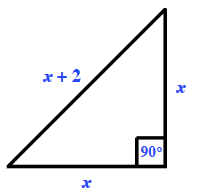### Home > PC > Chapter 7 > Lesson 7.2.1 > Problem7-38

7-38.

The hypotenuse of an isosceles right triangle is $2$ cm longer than the two legs. Find its area exactly.

1. Draw and label a diagram.
2. Use the Pythagorean Theorem to solve for $x$.
3. Then substitute the value found for $x$ into the area formula.